•5星
1KB qq_43045275 2021-05-20 17:09:55
•5星
623KB weixin_42685438 2021-09-29 06:19:54
• 708KB m0_52957036 2020-12-16 11:17:28
• 1.33MB cwjwhu 2013-11-12 21:10:43
• matlab实现图像处理频域滤波 傅里叶变换 含代码 matlab 快速傅立叶变换 离散时间傅立叶变换 图像处理

频域滤波步骤： 1.给定大小为M×NM\times NM×N的输入图像，将其填充为大小P×QP\times QP×Q的图像其中P=2M,Q=2NP=2M,Q=2NP=2M,Q=2N。填充方法为：在M,N的后面添加0（尾部加0）。设经过此步骤的图像为fp(x,y)f_p(x,...

频域滤波步骤：

1.给定大小为 M × N M\times N 的输入图像，将其填充为大小 P × Q P\times Q 的图像其中 P = 2 M , Q = 2 N P=2M,Q=2N 。填充方法为：在M,N的后面添加0（尾部加0）。设经过此步骤的图像为 f p ( x , y ) f_p(x,y)

进行第一步的原因（下面卷积公式应为f(m)）：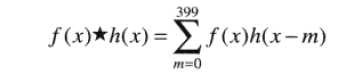如图，相当于对h(m)关于y轴对称之后向右不断平移x，并求其和f(m)的乘积。如果对称之后，h(m)的图像与h(x) or f(x)有重叠，就会产生混叠，影响结果。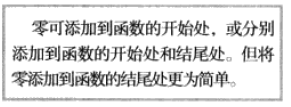2.对图像× ( − 1 ) x + y (-1)^{x+y} ，将其移到变换中心

进行第二步的原因：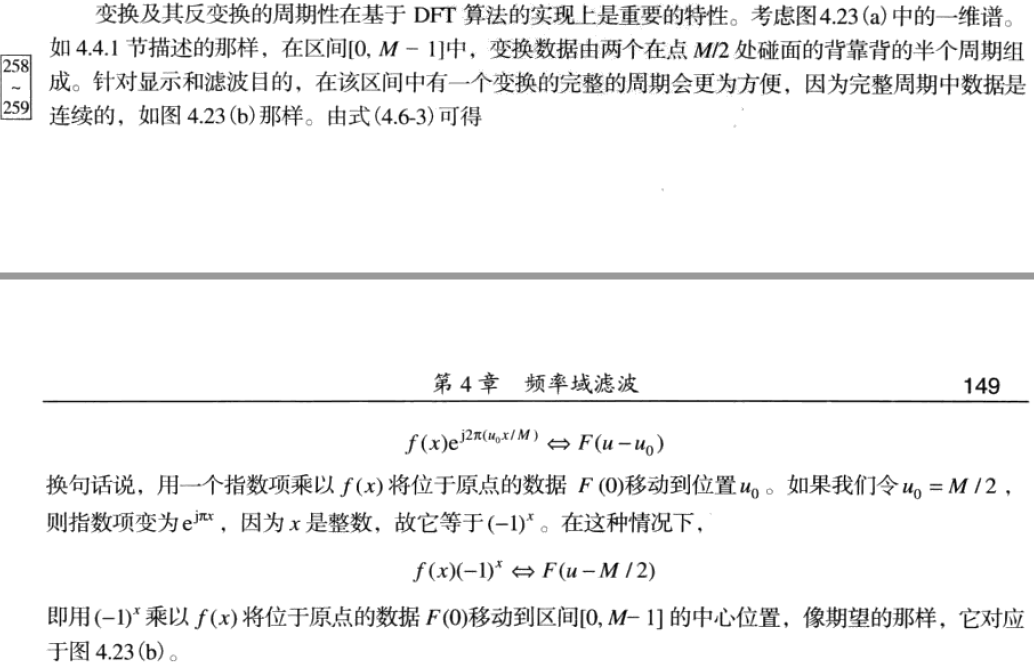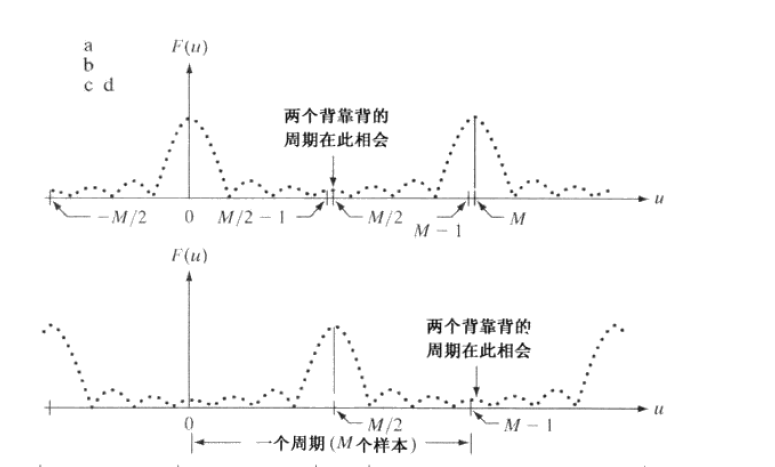如图，进行运算之后在 [ 0 , M − 1 ] [0,M-1] 内是完整的一个周期，便于DFT

3.计算步骤二之后获得的图像(矩阵)的DFT

就直接套公式就完事了
F ( u , v ) = ∑ x = 0 M − 1 ∑ y = 0 N − 1 f ( x , y ) e − 2 π j ( u x M + v y N ) F(u,v)=\sum_{x=0}^{M-1}\sum_{y=0}^{N-1}f(x,y)e^{-2\pi j(\frac{ux}{M}+\frac{vy}{N})}

4.生成一个实的，对称的滤波函数H(u,v)，其大小为P×Q，中心在(P/2,Q/2)处,G(u,v) = H(u,v).*F(u,v)

要注意滤波函数的生成大小，低通滤波器可以用

%D(u,v)<=D0时有值
u = 0:Q-1
v = 0:P-1
%calculate D(u,v)
H = double(D<=D0)

控制滤波器的大小

5.对G进行傅里叶反变换，得到g(x,y),并对其取实部，乘(-1)^(x+y)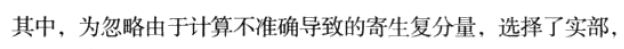其实取abs实际感觉差别不是很大

6.从g(x,y)上提取M*N的部分得到最终结果。

下面是代码实现

%matlab调库
function [DFT_img] = FFT_(I,H)
%调用FFT库函数实现频域滤波
%输入 I为图像f(x,y)，H为滤波器H(u,v)
%输出滤波后的图像g(x,y)
%在不严谨的情况下我们可以不做扩展0，结果仍然与正确结果差不多
F = fft2(I);
Fs = fftshift(F);
out = H.*Fs;
out=ifftshift(out);
out=ifft2(out);
out=real(out);%用abs基本没区别
out=out/max(out(:));%除以out中最大值
DFT_img = out;
end
%以低通滤波为例
M = size(I,2);
N = size(I,1);
u=0:M-1;
v=0:N-1;
[U,V]=meshgrid(u,v);%基于向量 x 和 y 中包含的坐标返回二维网格坐标
D=sqrt((U-M/2).^2+(V-N/2).^2);
D0=15;%截止频率
H=double(D<=D0);%理想低通滤波器
n = FFT_(I,H)
imshow(n,[])%不加[]效果相同

原图：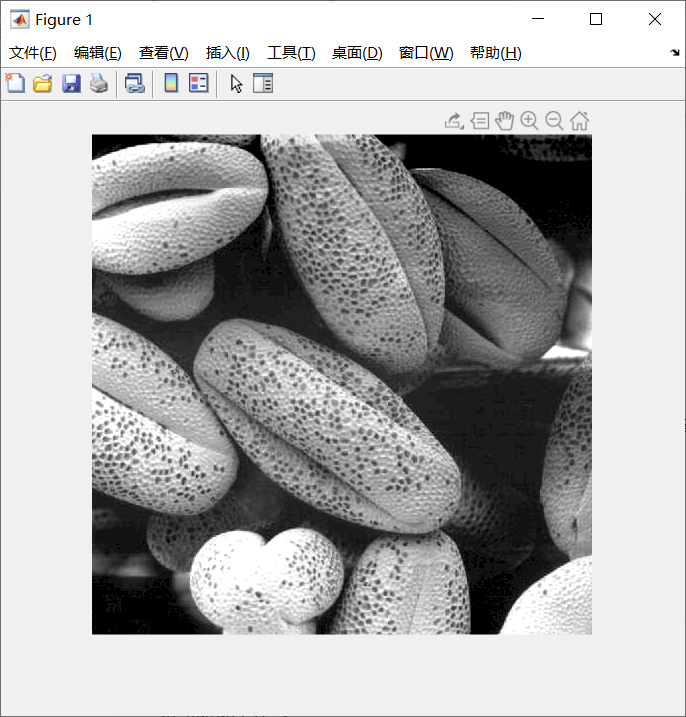低通滤波以后(D0=15)：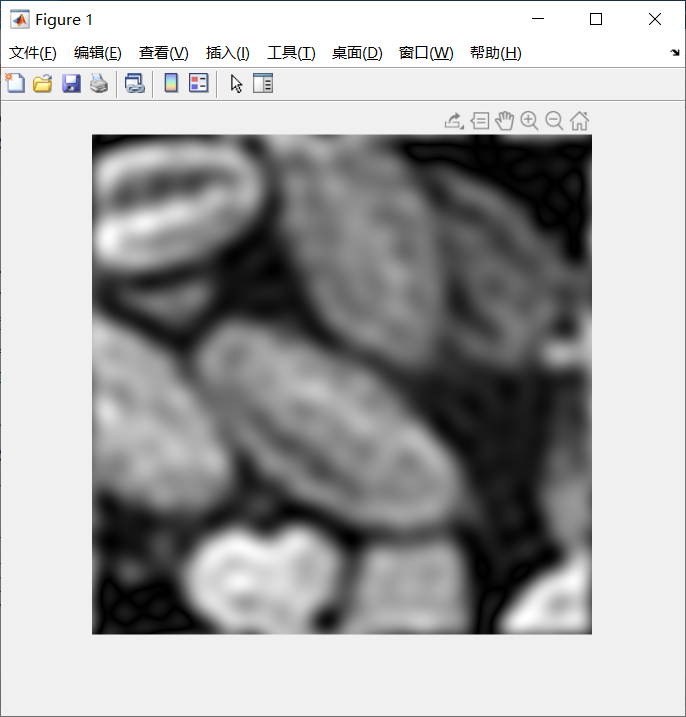若D0=5，可以看到更为模糊的图像：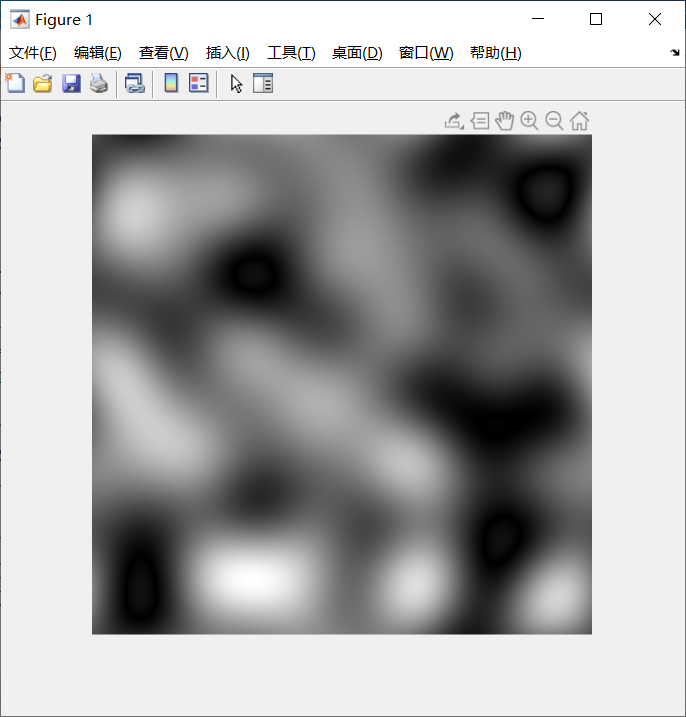matlab库函数解析：

1. Y = fft2(X)——输出X的二维傅里叶变换
Y = fft2(X,m,n) 将截断 X 或用尾随零填充 X，以便在计算变换之前形成 m×n 矩阵

2. fftshift(X)将零频分量移到屏幕中心，相当于我们上述讲的 × ( − 1 ) x + y \times(-1)^{x+y}
实际上fftshift操作的时候就是把矩阵X第一象限与第三象限交换，第二象限与第四象限交换。（相当于拆开再拼起来）

3. ifftshift(X) 撤销fftshift(X)的结果，实际还是第一象限与第三象限交换，第二象限与第四象限交换。

4. ifft2(X) 二维傅里叶逆变换

展开全文kid_a1412 2021-10-15 17:42:45
• 频率域法把图像看成一种二维信号进行二维傅里叶变换的信号增强，采用低通滤波法可以去掉图像的噪声；采用高通滤波法，则可增强边缘等高频信号，使模糊的图像变得清晰。滤波是一种重要的图像预处理手段，目前，...

摘要：图像的频域滤波是图像增强的一种方法。图像增强是图像处理的方法之一，有频率域法和空间域法。频率域法把图像看成一种二维信号，对其进行二维傅里叶变换的信号增强，采用低通滤波法可以去掉图像的噪声；采用高通滤波法，则可增强边缘等高频信号，使模糊的图像变得清晰。滤波是一种重要的图像预处理手段，目前，图像的频域滤波应用非常广泛，例如，基于频域滤波的织物疵点的检测、颤振信号处理中的时频域滤波方法等。

本文将主要研究常用图像滤波方法分析、频域滤波的特点及使用场合以及其如何用MATLAB实现图像的增强处理。

关键词：图像增强、频域滤波、MATLAB

目录

摘要

Abstract

1绪论-1

1.1数字图像处理的研究现状及发展方向-1

1.2研究内容和研究方法-2

1.2.1研究内容-2

1.2.2研究方法-2

2数字图像处理-2

2.1数字图像的基本概念-2

2.2数字图像处理软件-4

2.2.1 MATLAB简介-4

2.2.2其他图像处理软件的介绍-4

3图像的频率域变换-5

3.1连续傅里叶变换-5

3.1.1一维连续傅里叶变换-5

3.1.2二维连续傅里叶变换-6

3.2离散傅里叶变换-6

3.3快速傅里叶变换(FFT)-7

3.4本章小结-7

4图像增强-8

4.1图像噪声-8

4.2频率域图像增强概念-8

4.3 空间域滤波和频率域滤波的比较-9

5频率域滤波增强-9

5.1一些基本的滤波器介绍-10

5.2频率域低通滤波器-10

5.2.1理想低通滤波器-10

5.2.2巴特沃斯(Butterworth)低通滤波器-10

5.2.3高斯低通滤波器-11

5.3频率域高通滤波器-11

5.3.1理想高通滤波器-11

5.3.2巴特沃斯型高通滤波器-11

5.3.3高斯高通滤波器-12

5.4同态滤波器的简单介绍-12

5.5本章小结-12

6频域滤波增强应用实例-13

6.1理想型低通滤波器的应用-13

6.2巴特沃斯(Butterworth)型低通滤波器的应用-13

6.3高斯型低通滤波器的应用-15

6.4理想型高通滤波器的应用-16

6.5巴特沃斯型高通滤波器的应用-16

6.6高斯型高通滤波器的应用-17

6.7频域滤波应用于舰船热尾流红外图像-18

7结论-20

致谢-20

参考文献-21

附录-21

展开全文weixin_29299807 2021-04-22 11:19:17
• 2KB weixin_42696333 2021-09-10 21:09:51
• 基于matlab的傅里叶频域滤波 图像处理

865B qq_41050678 2018-05-10 01:12:22
• 提到滤波器，如果你首先想到的是巴特沃斯、切比雪夫等典型滤波器，以及通带宽度、阻带衰减、过渡带宽等关键参数，那么，你已经有一定的理论基础，甚至会设计软件滤波器解决数字信号频域滤波问题了。但是，本文讲到...

描述

滤波器，顾名思义，是将信号中一些特定频域的“波”滤除。按功能分类，可分为低通、高通、带通、带阻等。在现代各电子、电气系统中，可谓无处不在。

提到滤波器，如果你首先想到的是巴特沃斯、切比雪夫等典型滤波器，以及通带宽度、阻带衰减、过渡带宽等关键参数，那么，你已经有一定的理论基础，甚至会设计软件滤波器解决数字信号的频域滤波问题了。

但是，本文讲到的一些参数，却与这些完全不同，只有关注了这些参数，才能将滤波器理论基础转化为硬件实践。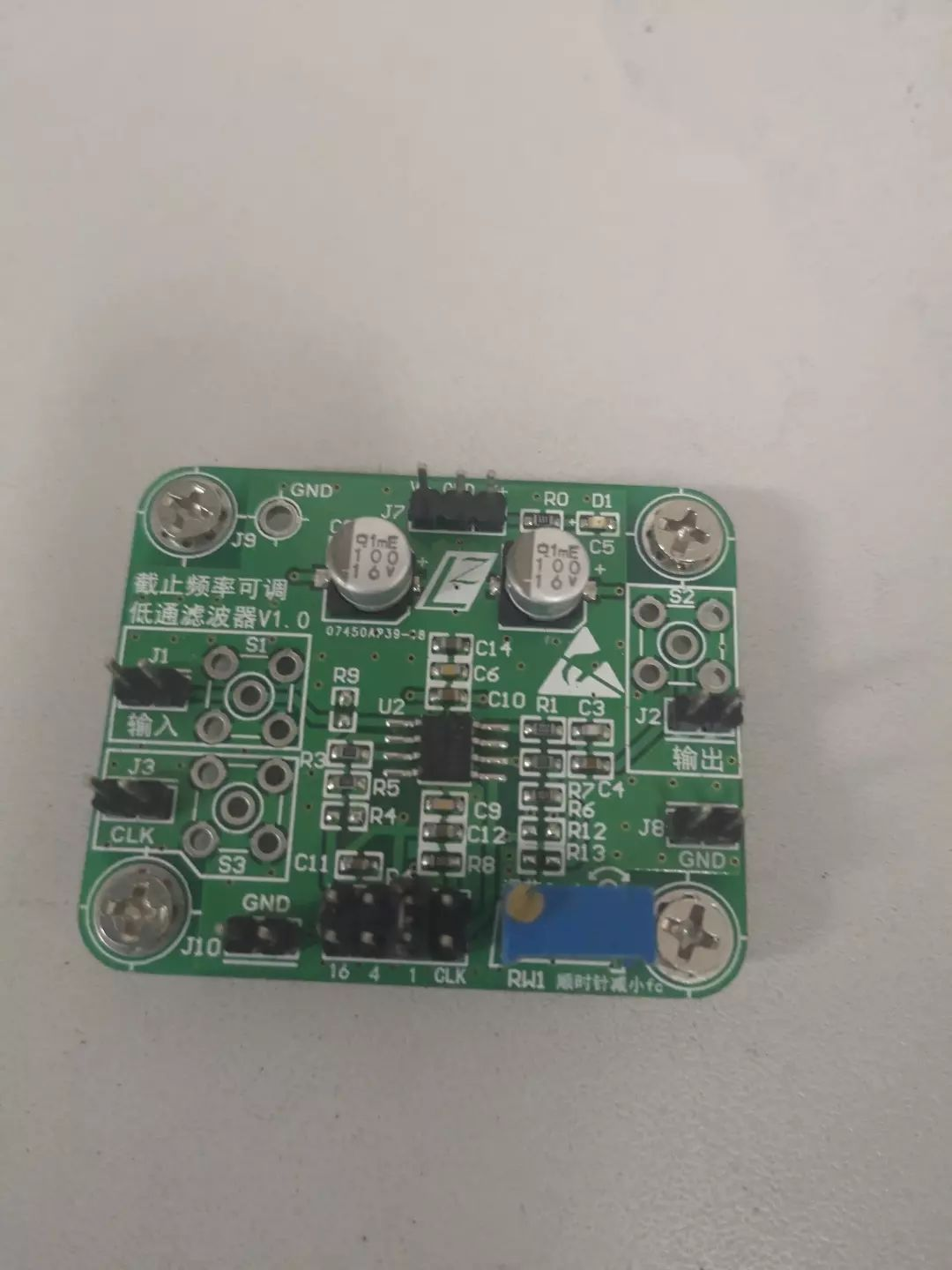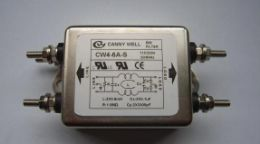一、灵敏度参数

灵敏度表征滤波器对元器件变化的敏感程度。把滤波器当做一个二端口网络，那么，其输出Vout与输入Vin存在关系：Vout=H(s)*Vin。这里，H(s)称为网络函数，或滤波器的传递函数。

而灵敏度S(x)，就是网络函数变化率与所关注元器件数值x偏差之间的比值，定义为：S(H,x)=(dH/H)/(dx/x)。

实际电路中，电阻、电容、电感都存在一定的误差，运放的放大系数、通带平坦度等也非绝对理想，而且随着温度、湿度等变化，元器件的数值也会发生变化，这些都会造成网络函数H(s)的变化，进而影响滤波效果。因此，在硬件滤波器设计中，一定要考虑元器件自身的精度。滤波器对关键元器件的灵敏度要足够低，元器件的精度应足够高。

二、输入阻抗与输出阻抗

信号源一般可等效为一个理想电压源和一个阻抗的串联。如果滤波器输入端与信号来源阻抗匹配，则能达到最大功率传输，如果滤波器输入为高阻，则能保持信号输入电压不变。而如果设计过程中未留意到输入阻抗这一参数，则会造成反射(高频)或分压(低频)，降低信号传输效率，造成信号衰减。

三、有源与无源滤波器

总的来说，低频信号滤波一般用有源滤波器，高频信号滤波一般用无源滤波器。

无源滤波器由无源R、L、C组成，在做低频信号的滤波器时，电感L的重量和体积都比较大，不利于滤波器的小型化、集成化，而且电感的使用也会使滤波效果没那么理想。

而有源滤波器一般不包含电感，由有源器件和R、C构成，重量轻且体积小，便于大规模生产，目前引起工程师的极大关注。但是有源RC滤波存在对高频特性的限制，一般运放的增益带宽积越大，成本也越高。所以处理高频信号时，有源滤波并不划算，相反无源滤波会起到较好效果。

四、元器件寄生参数

由于工艺、生产成本等等原因，市面上的元器件往往存在寄生参数。如：一般电阻、电容都有寄生电感(在直流供电时，稳压输出端一般并联10uF和0.1uF的旁路电容实现对高、低频噪声的滤波，这个0.1uF的旁路电容的作用主要就是寄生电感)。而空间分布的导线、元器件之间也存在分布电容。这些寄生参数会直接影响滤波器的各项参数。必要时，需要用无感电阻代替一般电阻。

总结

通过以上分析，不难看出：滤波器硬件设计并没有想象中那么简单。正如世界上没有两片相同的叶子，世界上也没有完全相同的两个滤波器。硬件实现过程中有太多的确定的或不确定的因素，会影响滤波结果。

因此，滤波器的设计不容易一步达成，往往需要不断调试，修改，再调试，再修改，复制性较差。这也造成一个多通道滤波器往往比多个单通道滤波器要贵很多。在这个领域，经验的积累比扎实的理论更宝贵。不过，如果你真正踏入这个领域，就会发现其中的无限乐趣和无穷魅力。

打开APP阅读更多精彩内容

点击阅读全文

展开全文weixin_42131405 2021-04-18 08:02:03
• Matlab之频域滤波（十五） matlab

基本概念就不概述了，我们都知道要进行滤波有空域和频域两种形式，而频域上的分析实际上就是进行傅里叶变换之后的结果的分析。而实际的图形中傅里叶变换之后所得中心会分布到图像四个角上，故就要进行频谱移中 这...

基本概念就不概述了，我们都知道要进行滤波有空域和频域两种形式，而频域上的分析实际上就是进行傅里叶变换之后的结果的分析。而实际的图形中傅里叶变换之后所得中心会分布到图像四个角上，故就要进行频谱移中

这部分是补充内容，具体可以结合这篇博客来学习一下
https://blog.csdn.net/qq_44790423/article/details/104740105

整体思路的实现代码为:

%假设原图大小为M×N
F = fft2(f);  %傅里叶变换
F = fft2(f, P, Q); %填充零使图像大小为P×Q
F1 = fftshift(F); %频谱移中
f2 = ifftshift(F1); %逆变换回空域再显示图像

故我们频域中的操作一般都是逆变换之前进行所需要的操作即可。

目录

频域滤波器

建立网格数组
function [U, V] = dftuv(M, N)
u = 0 : (M-1);
v = 0 : (N-1);
idx = find(u>M/2);
u(idx) = u(idx) - M;
idy = find(v >N/2);
v(idy) = v(idy) - N;
[V, U] = meshgrid(v, u);

[U, V] = dftuv(8,5);
D = U.^2 + V.^2
%计算矩阵中的每个点到原点的距离

可得到如下结果：

D =
0     1     4     4     1
1     2     5     5     2
4     5     8     8     5
9    10    13    13    10
16    17    20    20    17
9    10    13    13    10
4     5     8     8     5
1     2     5     5     2

低通滤波器
[h,w] = size(f);
[U,V] = dftuv(h, w);
D0 = 0.5*w; %取截止频率为图片宽度的0.5
F = fftshift(fft2(f, h, w)); %转换为频域再用fftshift进行平移
H = exp(-(U.^2 + V.^2)/(2*(D0^2)));
G = F.*H;   %频域内滤波
g = ifft2(ifftshift(G)); %回到空域
g = g(1: size(f, 1), 1: size(f, 2)); %截取图片出来

figure
subplot(121)
imshow(fftshift(H), []);

subplot(122)
imshow(log(1+abs(F)), []);

figure
subplot(121)
imshow(f)
subplot(122)
imshow(g);

下图为低通滤波器和移中后的频域波形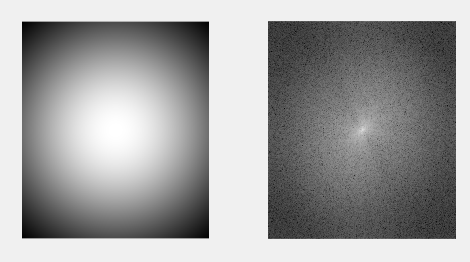下图为原图和经低通滤波器的波形（只可以看到图形的大致轮廓）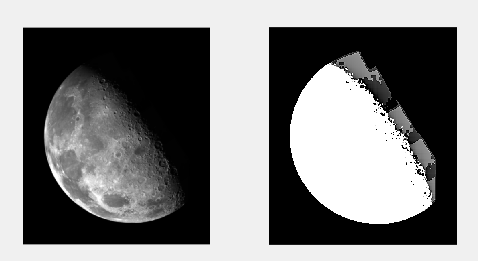绘制线框图和表面图

一般使用函数mesh

线框图mesh
mesh(H)
%绘制一个x=1:M 和y= 1:N的线框图，其中[M,N]=size(H)

该若M和N很大，则线框图的密集程度会大到不可接受，在这种情况下，我们可以使用语法

mesh(H(1:k:end，1:k:end))
%绘制第k个点
%沿每个轴4060个细分可在外观与分辨率之间提供了较好的平衡

colormap
%绘制彩色的线
colormap([0 0 0]) %设为黑色

补充：

grid off %关闭网格
axis off %关闭坐标轴
view(az, e1) %控制观测位置，az为方位角，e1为仰角

举个例子：

[h,w] = size(f)
[U,V] = dftuv(h, w);
D0 = 50; %取截止频率为图片宽度的0.5
F = fftshift(fft2(f, h, w)); %转换为频域再用fftshift进行平移
H = exp(-(U.^2 + V.^2)/(2*(D0^2)));
H = fftshift(H);

subplot(221)
mesh(H(1:10:540, 1:10:466))
axis([0 48 0 50 0 1])

subplot(222)
mesh(H(1:10:540, 1:10:466))
colormap([0 0 1])

subplot(223)
mesh(H(1:10:540, 1:10:466))
grid off
axis off

subplot(224)
mesh(H(1:10:540, 1:10:466))
grid off
axis off
view(-25, 0)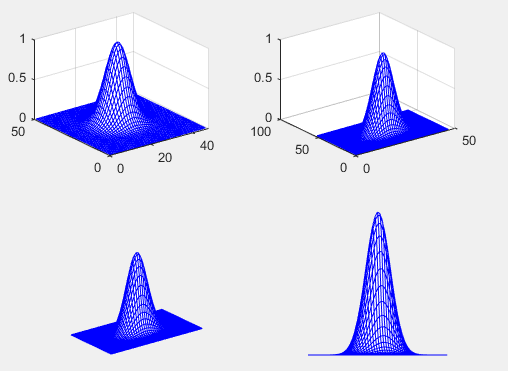表面图

一般用surf

[Y X] = meshgrid(-2:0.1:2, -2:0.1:2);
Z = X.*exp(-X.^2 - Y.^2);
subplot(121)
mesh(Z)
subplot(122)
surf(Z)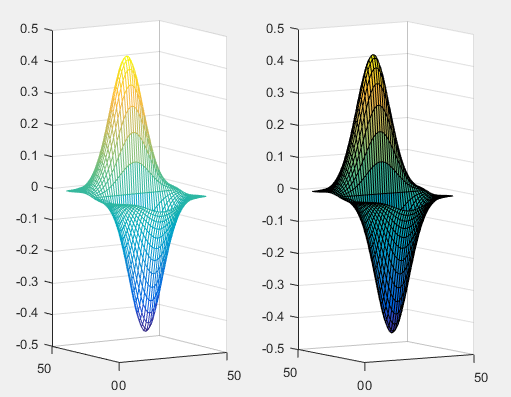再对图二进行一个操作

subplot(122)
surf(Z)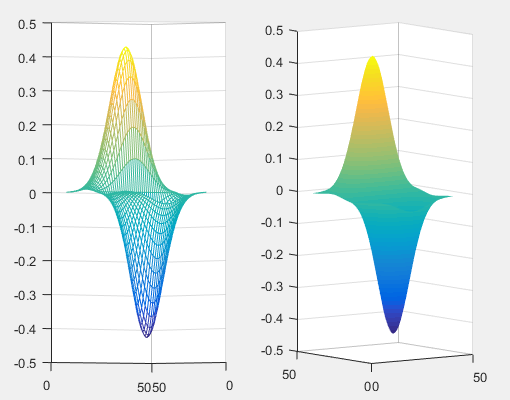高通滤波器
[h,w] = size(f);
[U,V] = dftuv(h, w);
D0 = 80;
F = fftshift(fft2(f, h, w)); %转换为频域再用fftshift进行平移
H = 1 - exp(-(U.^2 + V.^2)/(2*(D0^2)));
G = F.*H;   %频域内滤波
g = ifft2(ifftshift(G)); %回到空域
g = g(1: size(f, 1), 1: size(f, 2)); %截取图片出来

figure
subplot(121)
imshow(fftshift(H), []);

subplot(122)
imshow(log(1+abs(F)), []);

figure
subplot(121)
imshow(f)
subplot(122)
imshow(g);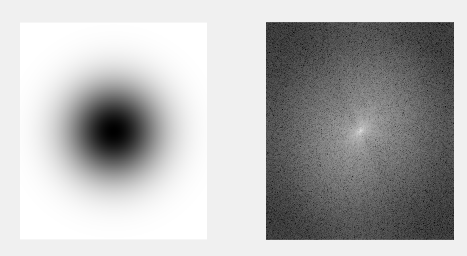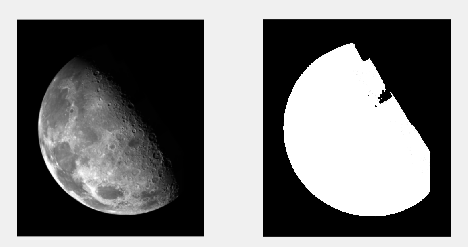同低通滤波器一样，只是用1减去低通就可得到，所以原理也很简单呢！只是补充了一些小东西

展开全文qq_44790423 2020-04-15 16:57:50
• weixin_31495827 2021-04-19 07:21:50
• 图像处理：频域滤波_Matlab matlab 频域滤波 数字图像 图像处理 冈萨雷斯

tyfwin 2019-05-07 16:05:53
• xiong_xin 2020-04-06 19:42:33
• 基于matlab GUI空域+频域滤波图像去噪 matlab

m0_60115601 2021-07-19 15:08:55
• 冈萨雷斯数字图像处理-数字图像基础（Matlab）-图像的频域滤波 冈萨雷斯数字图像处理 图像的频域滤波 Matlab 高斯滤波

u010936286 2018-05-13 20:39:33
• 19.32MB weixin_43907374 2018-12-02 17:47:25
• u010637291 2020-05-27 18:53:45
• matlab：高斯函数对图像的空域滤波和频域滤波 matlab 图像处理

qq_39297053 2021-03-08 13:20:23
• 3KB weixin_38532629 2021-05-22 14:54:20
• 使用 Matlab 实现的信号分析：用于在时域、频域和时频域中进行信号分析的 GUI。-matlab开发 matlab

2.2MB weixin_38518885 2021-06-01 01:16:34
• 7KB chenshuo_szu 2014-11-26 12:25:10
• 灰度图像的频域滤波：灰度图像频域滤波器的简单Matlab实现-matlab开发 matlab

319KB weixin_38717843 2021-05-30 14:56:23
• weixin_33397740 2021-04-24 23:57:01
• Matlab 实现信号滤波 matlab filter 信号处理

qq_36758914 2020-11-30 10:07:26
• Matlab频域滤波一些总结 图像处理

weixin_40883324 2018-05-15 09:45:12
•5星
1KB qq_43045275 2021-05-20 17:09:55
• 基本空域滤波,空域滤波和频域滤波,matlab源码 空域滤波

1.66MB weixin_42696333 2021-09-10 17:05:06
• Matlab实现频域滤波——二维傅里叶变换、低通、高通 matlab

maggieyiyi 2021-08-26 11:24:00
• 基于MATLAB的空间滤波与频域滤波 matlab

A5555564321 2015-09-15 15:24:46
• m0_52357468 2021-03-06 20:45:22
•5星
3.92MB qdmzy1 2012-04-21 16:18:15...

matlab对信号进行频域滤波matlab 订阅